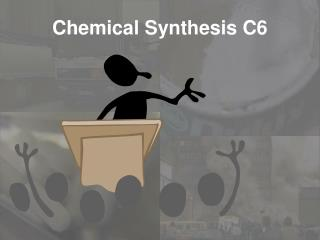DownloadDownload PresentationChemical Synthesis C6

# Chemical Synthesis C6

Télécharger la présentation## Chemical Synthesis C6

- - - - - - - - - - - - - - - - - - - - - - - - - - - E N D - - - - - - - - - - - - - - - - - - - - - - - - - - -
##### Presentation Transcript

1. Chemical Synthesis C6

2. H2SO4 • Name? • Name of a salt you can make from it.

3. 3. Give the formula Carbon dioxide

4. 4. Give the formula Sulphuric acid

5. 5. Give the formula Calcium chloride

6. 6. Which are solids? • Hydrogen chloride • Sulfuric acid • Nitric acid • Ethanoic acid • Tartaric acid • Citric acid

7. 7. What is an indicator?8. What is neutralisation?9. What does this mean?

8. 10. Acid + metal  ? + ?11. Acid + metal oxide  ? + ?12. Acid + metal hydroxide  ? + ?13. Acid + metal carbonate  ? + ? + ?

9. 14. What’s the salt? Sulfuric acid Magnesium

10. 15. What’s the salt? Nitric acid Copper oxide

11. 16. Name the apparatus

12. 17. 4 ways to speed up a reaction ..

13. 18. Why does a reaction go faster when the acid is more concentrated? • Particles • crowded • collisions • frequent

14. 19. What do you know about catalysts?

15. Mass lost (g) 20oC 10oC Time (min) 20. What does this tell you?

16. 21. Why are rates important in industry?

17. 23. Relative formula mass. This tells you the number of times a molecule is heavier than an atom of hydrogen E.g. Work out the Mr of CO2.

18. 24.The recipe bit … What mass of ammonia is produced from 14g of nitrogen? Underline N2 + 3H2 2NH3 Molecules in x relative formula mass Same at both sides Keep the same units!

19. 25. Percentage yield You expected 100 tonnes of ammonium sulphate but the actual mass was 25 tonnes. What was the % yield?

20. Good luck!